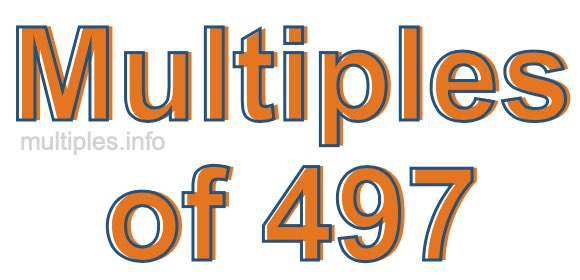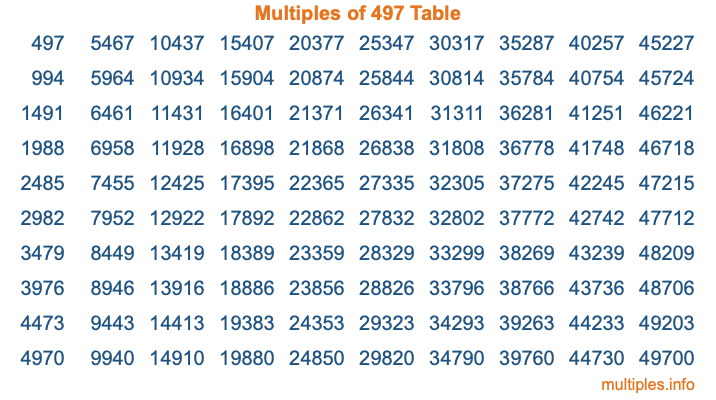Multiples of 497Welcome to the Multiples of 497 page. Here we will first teach you everything you will ever need to know about the multiples of 497, and then give you a study guide summary of everything we taught you to make sure you remember it all. Use this page to look up facts and learn information about the multiples of 497. This page will make you a multiples of four hundred ninety-seven expert!

Definition of Multiples of 497
Multiples of 497 are all the numbers that when divided by 497 equal an integer. Each of the multiples of 497 are called a multiple. A multiple of 497 is created by multiplying 497 by an integer.

Therefore, to create a list of multiples of 497, you start with 1 multiplied by 497, then 2 multiplied by 497, then 3 multiplied by 497, and so on for as long as you want. Thus, the list of the first five multiples of 497 is 497, 994, 1491, 1988, and 2485. To see a larger list of multiples of 497, see the printable image of Multiples of 497 further down on this page. We also have a category where you can choose any nth multiple of 497.

Multiples of 497 Checker
The Multiples of 497 Checker below checks to see if any number of your choice is a multiple of 497. In other words, it checks to see if there is any number (integer) that when multiplied by 497 will equal your number. To do that, we divide your number by 497. If the the quotient is an integer, then your number is a multiple of 497.

Is  a multiple of 497?

Least Common Multiple of 497 and ...
A Least Common Multiple (LCM) is the lowest multiple that two or more numbers have in common. This is also called the smallest common multiple or lowest common multiple and is useful to know when you are adding our subtracting fractions. Enter one or more numbers below (497 is already entered) to find the LCM.

Check out our LCM Calculator if you need more details about the Least Common Multiple or if you need the LCM for different numbers for adding and subtraction fractions.

nth Multiple of 497
As we stated above, 497 is the first multiple of 497, 994 is the second multiple of 497, 1491 is the third multiple of 497, and so on. Enter a number below to find the nth multiple of 497.

th multiple of 497

Multiples of 497 vs Factors of 497
497 is a multiple of 497 and a factor of 497, but that is where the similarities end. All postive multiples of 497 are 497 or greater than 497. All positive factors of 497 are 497 or less than 497.

Below is the beginning list of multiples of 497 and the factors of 497 so you can compare:

Multiples of 497: 497, 994, 1491, 1988, 2485, etc.

Factors of 497: 1, 7, 71, 497

As you can see, the multiples of 497 are all the numbers that you can divide by 497 to get a whole number. The factors of 497, on the other hand, are all the whole numbers that you can multiply by another whole number to get 497.

It's also interesting to note that if a number (x) is a factor of 497, then 497 will also be a multiple of that number (x).

Multiples of 497 vs Divisors of 497
The divisors of 497 are all the integers that 497 can be divided by evenly. Below is a list of the divisors of 497.

Divisors of 497: 1, 7, 71, 497

The interesting thing to note here is that if you take any multiple of 497 and divide it by a divisor of 497, you will see that the quotient is an integer.

Multiples of 497 Table
Below is an image of the first 100 multiples of 497 in a table. The table is in chronological order, column by column. The first column has the first ten multiples of 497, the second column has the next ten multiples of 497, and so on.The Multiples of 497 Table is also referred to as the 497 Times Table or Times Table of 497. You are welcome to print out our table for your studies.

Negative Multiples of 497
Although not often discussed or needed in math, it is worth mentioning that you can make a list of negative multiples of 497 by multiplying 497 by -1, then by -2, then by -3, and so on, to get the following list of negative multiples of 497:

-497, -994, -1491, -1988, -2485, etc.

Multiples of 497 Summary
Below is a summary of important Multiples of 497 facts that we have discussed on this page. To retain the knowledge on this page, we recommend that you read through the summary and explain to yourself or a study partner why they hold true.

There are an infinite number of multiples of 497.

A multiple of 497 divided by 497 will equal a whole number.

497 divided by a factor of 497 equals a divisor of 497.

The nth multiple of 497 is n times 497.

The largest factor of 497 is equal to the first positive multiple of 497.

497 is a multiple of every factor of 497.

497 is a multiple of 497.

A multiple of 497 divided by a divisor of 497 equals an integer.

497 divided by a divisor of 497 equals a factor of 497.

Any integer times 497 will equal a multiple of 497.

Multiples of a Number
Here you can get the multiples of another number, all with the same attention to detail as we did for multiples of 497 on this page.

Multiples of
Multiples of 498
Did you find our page about multiples of four hundred ninety-seven educational? Do you want more knowledge? Check out the multiples of the next number on our list!